## RF Designer - Components (Physical Transmission Lines)

Full listing and description of components that can be placed in an RF Designer schematic.
rftools
Posts: 13
Joined: Thu May 22, 2014 12:36 pm

### RF Designer - Components (Physical Transmission Lines)

Physical Transmission Line Elements

TLINP - Physical Transmission Line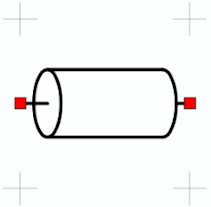Name Description Units Default Value Z Impedance Resistance 50 ohms L Physical length Dimensions 1000 mil εeff Effective dielectric constant Unitless 1.0 A Attenuation per unit length decibels 0 dB/mil F0 Frequency for attenuation factor Frequency 1000 MHz

Model for a physical and lossy transmission line. Electrical length of the transmission line is calculated at each simulated frequency using the user supplied physical length (L) and the effective dielectric constant (Eeff).
Loss per unit length is determined by the user supplied loss (A) and frequency for scaling (F0). If F0 is set to 0, then the loss per unit length is constant regardless of simulation frequency. Otherwise, the loss is scaled by the square root of the ratio of the simulation frequency and F0 (sqrt(f/F0)).

TLPOC - Physical Open Circuited Transmission Line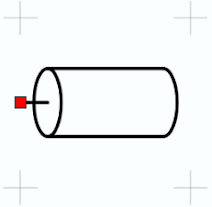Name Description Units Default Value Z Impedance Resistance 50 ohms L Physical length Dimensions 1000 mil εeff Effective dielectric constant Unitless 1.0 A Attenuation per unit length decibels 0 dB/mil F0 Frequency for attenuation factor Frequency 1000 MHz

Model for a physical and lossy open circuited transmission line. Electrical length of the transmission line is calculated at each simulated frequency using the user supplied physical length (L) and the effective dielectric constant (Eeff).
Loss per unit length is determined by the user supplied loss (A) and frequency for scaling (F0). If F0 is set to 0, then the loss per unit length is constant regardless of simulation frequency. Otherwise, the loss is scaled by the square root of the ratio of the simulation frequency and F0 (sqrt(f/F0)).

TLPSC - Physical Short Circuited Transmission Line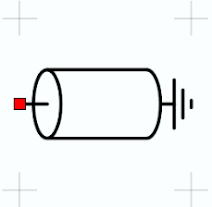Name Description Units Default Value Z Impedance Resistance 50 ohms L Physical length Dimensions 1000 mil εeff Effective dielectric constant Unitless 1.0 A Attenuation per unit length decibels 0 dB/mil F0 Frequency for attenuation factor Frequency 1000 MHz

Model for a physical and lossy short circuited transmission line. Electrical length of the transmission line is calculated at each simulated frequency using the user supplied physical length (L) and the effective dielectric constant (Eeff).
Loss per unit length is determined by the user supplied loss (A) and frequency for scaling (F0). If F0 is set to 0, then the loss per unit length is constant regardless of simulation frequency. Otherwise, the loss is scaled by the square root of the ratio of the simulation frequency and F0 (sqrt(f/F0)).

TLINP4 - Physical 4 Node Transmission Line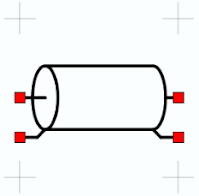Name Description Units Default Value Z Impedance Resistance 50 ohms L Physical length Dimensions 1000 mil εeff Effective dielectric constant Unitless 1.0 A Attenuation per unit length decibels 0 dB/mil F0 Frequency for attenuation factor Frequency 1000 MHz

Model for a physical and lossy transmission line with the ground terminals left open and available as ports in the schematic. Electrical length of the transmission line is calculated at each simulated frequency using the user supplied physical length (L) and the effective dielectric constant (Eeff).
Loss per unit length is determined by the user supplied loss (A) and frequency for scaling (F0). If F0 is set to 0, then the loss per unit length is constant regardless of simulation frequency. Otherwise, the loss is scaled by the square root of the ratio of the simulation frequency and F0 (sqrt(f/F0)).

CLINP - Physical Coupled Transmission Line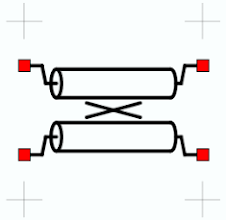Name Description Units Default Value Zoe Even-mode impedance Resistance 69 ohms Zoo Odd-mode impedance Resistance 36 ohms L Physical length Dimensions 1000 mil εeffE Even-mode effective dielectric constant Unitless 1.0 εeffO Odd-mode effective dielectric constant Unitless 1.0 AE Even-mode attenuation per unit length decibels 0 dB/mil AO Odd-mode attenuation per unit length decibels 0 dB/mil

Model for a pair of lossy symmetric coupled transmission lines. Length is specified as a physical length(L). Coupling is determined by the even and odd mode impedances (Zoe and Zoo), effective dielectrics (EeffE and EeffO), and attenuation per unit length(AE and AO) over the specified physical length (L).

Given a desired coupling C in decibels, Zoe = Zo * ((1 + k) / (1 - k)) where k = power(10.0, -C/20.0). Zoo can the be found by solving Zoo = Zo * ((1 - k) / (1 + k))

Example: Find Zoe and Zoo for a 10 dB coupler in a 50 ohm system.
First calculate k as k = power(10.0, -10/20) = 0.316
Zoe is found as Zoe = Zo * ((1 + .316) / (1 - .316)) = 96.2 ohms
Zoo is found as Zoo = Zo * ((1 - .316) / (1 + .316)) = 26 ohms